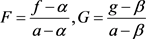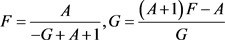﻿ 整函数与其差分多项式的唯一性

整函数与其差分多项式的唯一性Uniqueness of Entire Functions That Share Small Function with Their Difference Polynomials

Abstract: In this paper, we study the uniqueness of difference operators about transcendental entire function f(z) with a Borel entire exceptional function, which shares a small function a(z) with its difference polynomial. Furthermore, under the above assumption, we replace the condition “Borel entire exceptional function” by “δ(0,f)＞0”，and get the same result when f(z) shares a(z) CM with its difference polynomial.

1. 引言

$\begin{array}{c}\stackrel{¯}{N}\left(r,\frac{1}{F-1}\right)={\stackrel{¯}{N}}_{E}^{1\right)}\left(r,\frac{1}{F-1}\right)+{\stackrel{¯}{N}}_{L}\left(r,\frac{1}{F-1}\right)+{\stackrel{¯}{N}}_{L}\left(r,\frac{1}{G-1}\right)+{\stackrel{¯}{N}}_{E}^{\left(2}\left(r,\frac{1}{G-1}\right)\\ =\stackrel{¯}{N}\left(r,\frac{1}{G-1}\right)\end{array}$

$g\left(z\right)={m}_{1}\left(z\right)f\left(z+{c}_{1}\right)+{m}_{2}\left(z\right)f\left(z+{c}_{2}\right)+\cdots +{m}_{k}\left(z\right)f\left(z+{c}_{k}\right)$

$g\left(z\right)={m}_{1}\left(z\right)f\left(z+{c}_{1}\right)+{m}_{2}\left(z\right)f\left(z+{c}_{2}\right)+\cdots +{m}_{k}\left(z\right)f\left(z+{c}_{k}\right)$

$g\left(z\right)={m}_{1}\left(z\right)f\left(z+{c}_{1}\right)+{m}_{2}\left(z\right)f\left(z+{c}_{2}\right)+\cdots +{m}_{k}\left(z\right)f\left(z+{c}_{k}\right)$

2. 几个引理

$m\left(r,\frac{f\left(z+c\right)}{f\left(z\right)}\right)=S\left(r,f\right)$,

$N\left(r,\frac{1}{{f}^{\left(k\right)}}\right)\le N\left(r,\frac{1}{f}\right)+kN\left(r,f\right)+S\left(r,f\right)$.

${N}_{E}{}^{1\right)}\left(r,\frac{1}{F-1}\right)\le N\left(r,H\right)+S\left(r,F\right)+S\left(r,G\right)$.

$\lambda \left(f\cdot g\right)\le \mathrm{max}\left\{\lambda \left(f\right),\lambda \left(g\right)\right\}$.

$T\left(r,f\right)=o\left(T\left(r,g\right)\right)$.

$\lambda \left(H\right)<\lambda \left(f-\alpha \right)=\lambda \left(f\right)\le \mathrm{max}\left\{\lambda \left(H\right),\lambda \left({e}^{Q}\right)\right\}=\lambda \left({e}^{Q}\right)$,

$\lambda \left({e}^{Q}\right)\le \mathrm{max}\left\{\lambda \left(H\right),\lambda \left(f\right)\right\}=\lambda \left(f\right)$,

$\lambda \left(f\right)=\lambda \left({e}^{Q}\right)=\mu \left({e}^{Q}\right)$。又由引理5可得。因此

$\begin{array}{c}T\left(r,f\right)=T\left(r,f-\alpha \right)+S\left(r,f\right)\\ =T\left(r,H{e}^{Q}\right)+S\left(r,f\right)\\ \le T\left(r,H\right)+T\left(r,{e}^{Q}\right)+S\left(r,f\right)\\ \le T\left(r,{e}^{Q}\right)+S\left(r,f\right)+S\left(r,{e}^{Q}\right)\end{array}$,

$\delta \left(\alpha ,f\right)=1-\stackrel{¯}{\underset{r\to \infty }{\mathrm{lim}}}\frac{N\left(r,\frac{1}{f-\alpha }\right)}{T\left(r,f\right)}\le 1-\stackrel{¯}{\underset{r\to \infty }{\mathrm{lim}}}\frac{N\left(r,\frac{1}{H}\right)}{T\left(r,{e}^{Q}\right)}=1$.

3. 定理1的证明, (1)

$T\left(r,g\right)\le T\left(r,f\right)+S\left(r,f\right)=m\left(r,\frac{1}{f-\alpha }\right)+S\left(r,f\right)\le m\left(r,\frac{g-\beta }{f-\alpha }\right)+m\left(r,\frac{1}{g-\beta }\right)+S\left(r,f\right)$,

$T\left(r,g\right)=m\left(r,g\right)+S\left(r,f\right)$。故 $N\left(r,\frac{1}{g-\beta }\right)=S\left(r,f\right)$。从而有 $N\left(r,\frac{1}{F}\right)=S\left(r,f\right)$$N\left(r,\frac{1}{G}\right)=S\left(r,f\right)$

$\begin{array}{c}N\left(r,H\right)\le {\stackrel{¯}{N}}_{\left(2}\left(r,\frac{1}{F}\right)+{\stackrel{¯}{N}}_{\left(2}\left(r,\frac{1}{G}\right)+{N}_{L}\left(r,\frac{1}{F-1}\right)\\ \text{\hspace{0.17em}}\text{\hspace{0.17em}}+{N}_{L}\left(r,\frac{1}{G-1}\right)+{N}_{0}\left(r,\frac{1}{{F}^{\prime }}\right)+{N}_{0}\left(r,\frac{1}{{G}^{\prime }}\right)\\ \le {N}_{L}\left(r,\frac{1}{F-1}\right)+{N}_{L}\left(r,\frac{1}{G-1}\right)+{N}_{0}\left(r,\frac{1}{{F}^{\prime }}\right)+{N}_{0}\left(r,\frac{1}{{G}^{\prime }}\right)\end{array}$, (2). (3). (4)

$\begin{array}{l}\stackrel{¯}{N}\left(r,\frac{1}{F-1}\right)+\stackrel{¯}{N}\left(r,\frac{1}{G-1}\right)\\ ={\stackrel{¯}{N}}_{E}^{1\right)}\left(r,\frac{1}{F-1}\right)+3{N}_{L}\left(r,\frac{1}{F-1}\right)+3{N}_{L}\left(r,\frac{1}{G-1}\right)\\ \text{\hspace{0.17em}}\text{\hspace{0.17em}}\text{ }\text{ }+2{N}_{E}^{\left(2}\left(r,\frac{1}{G-1}\right)+{N}_{0}\left(r,\frac{1}{{F}^{\prime }}\right)+{N}_{0}\left(r,\frac{1}{{G}^{\prime }}\right)+S\left(r,f\right)\end{array}$. (5)

${N}_{L}\left(r,\frac{1}{F-1}\right)+2{N}_{L}\left(r,\frac{1}{G-1}\right)+{N}_{E}^{1\right)}\left(r,\frac{1}{F-1}\right)+2{N}_{E}^{\left(2}\left(r,\frac{1}{G-1}\right)\le N\left(r,\frac{1}{G-1}\right)\le T\left(r,G\right)$. (6)

$\begin{array}{c}\stackrel{¯}{N}\left(r,\frac{1}{F-1}\right)+\stackrel{¯}{N}\left(r,\frac{1}{G-1}\right)\le 2{N}_{L}\left(r,\frac{1}{F-1}\right)+{N}_{L}\left(r,\frac{1}{G-1}\right)+T\left(r,G\right)\\ \text{\hspace{0.17em}}\text{\hspace{0.17em}}+{N}_{0}\left(r,\frac{1}{{F}^{\prime }}\right)+{N}_{0}\left(r,\frac{1}{{G}^{\prime }}\right)+S\left(r,f\right)\end{array}$. (7)

$T\left(r,F\right)\le 2{N}_{L}\left(r,\frac{1}{F-1}\right)+{N}_{L}\left(r,\frac{1}{G-1}\right)+S\left(r,f\right)$. (8)

$\begin{array}{c}2{N}_{L}\left(r,\frac{1}{F-1}\right)+{N}_{L}\left(r,\frac{1}{G-1}\right)\le 2N\left(r,\frac{1}{{F}^{\prime }}\right)+N\left(r,\frac{1}{{G}^{\prime }}\right)\\ \le 2N\left(r,\frac{1}{F}\right)+N\left(r,\frac{1}{G}\right)\\ =S\left(r,f\right)\end{array}$. (9)

$\frac{1}{F-1}=\frac{A}{G-1}+B$, (10)

$F=\frac{\left(B+1\right)G+A-B-1}{BG+A-B},G=\frac{\left(B-A\right)F+A-B-1}{BF-B-1}$. (11)

$\begin{array}{c}T\left(r,f\right)=T\left(r,F\right)+S\left(r,f\right)\\ \le \stackrel{¯}{N}\left(r,\frac{1}{F}\right)+\stackrel{¯}{N}\left(r,\frac{1}{F-\frac{B+1}{B}}\right)+S\left(r,f\right)\\ =\stackrel{¯}{N}\left(r,G\right)+S\left(r,f\right)=S\left(r,f\right)\end{array}$, (12)

$T\left(r,f\right)=S\left(r,f\right)$。矛盾。. (13)

$A\ne 1$，从(13)可得 $N\left(r,\frac{1}{F-\frac{A-1}{A}}\right)=N\left(r,G\right)$。类似于情形1的证明过程我们同样可得矛盾。因此 A=1 。由(10)可知 $F\equiv G$，即 $f\equiv g$. (14)

$A\ne -1$，从(14)可得 $F\cdot G\equiv 1$，即

$\left(f-\alpha \right)\left(g-\beta \right)\equiv \left(a-\alpha \right)\left(a-\beta \right)$. (15)

$\begin{array}{c}2T\left(r,f\right)=2T\left(f-\alpha \right)+S\left(r,f\right)=T\left(r,\frac{1}{{\left(f-\alpha \right)}^{2}}\right)+S\left(r,f\right)\\ =m\left(r,\frac{1}{{\left(f-\alpha \right)}^{2}}\right)+N\left(r,\frac{1}{{\left(f-\alpha \right)}^{2}}\right)+S\left(r,f\right)\\ \le m\left(r,\frac{g-\beta }{f-\alpha }\frac{1}{\left(f-\alpha \right)\left(g-\beta \right)}\right)+S\left(r,f\right)\\ \le m\left(r,\frac{g-\beta }{f-\alpha }\right)+m\left(r,\frac{1}{\left(a-\alpha \right)\left(a-\beta \right)}\right)+S\left(r,f\right)\\ \le T\left(r,a-\alpha \right)+T\left(r,a-\beta \right)+S\left(r,f\right)=S\left(r,f\right)\end{array}$. (16)

$T\left(r,f\right)=S\left(r,f\right)$。矛盾。因此定理1得证。

4. 定理2的证明

$\frac{g-a}{f-a}={e}^{H}$, (17)

$g-a={e}^{H}\left(f-a\right)-\left(f-a\right)+f-a=\left({e}^{H}-1\right)\left(f-a\right)+f-a$,

$g=\left({e}^{H}-1\right)\left(f-a\right)+f$. (18)

$\frac{g}{\left({e}^{H}-1\right)fa}=\frac{f-a}{fa}+\frac{1}{\left({e}^{H}-1\right)a}=\frac{1}{a}-\frac{1}{f}+\frac{1}{\left({e}^{H}-1\right)a}$,$\begin{array}{c}m\left(r,\frac{1}{f}\right)=m\left(r,\frac{1}{\left({e}^{H}-1\right)a}+\frac{1}{a}-\frac{g}{\left({e}^{H}-1\right)fa}\right)\\ \le 3m\left(r,\frac{1}{a}\right)+2m\left(r,\frac{1}{{e}^{H}-1}\right)+m\left(r,\frac{g}{f}\right)+O\left(1\right)\\ \le 2m\left(r,\frac{1}{{e}^{H}-1}\right)+S\left(r,f\right)\end{array}$. (19)

$T\left(r,{e}^{H}\right)\le N\left(r,\frac{1}{{e}^{H}-1}\right)+S\left(r,{e}^{H}\right)\le T\left(r,{e}^{H}\right)+S\left(r,{e}^{H}\right)$,

 Yang, L. (1993) Value Distribution Theory. Springer-Verlag, Berlin

 Yang, C.C. and Yi, H.X. (2003) Uniqueness Theory of Meromorphic Functions. Kluwer Academic Publishers Group, Dordrecht.

 Huang, Z.B. and Zhang, R.R. (2018) Uniqueness of the Difference of Meromorphic Functions. Analysis Mathematica, 44, 461-473.

 Heittokangas, Korhonen, R., Laine, I. and Rieppo, J. (2011) Uniqueness of Meromorphic Functions Sharing Values with Their Shifts. Complex Variables and Elliptic Equations, 56, 81-92.
https://doi.org/10.1080/17476930903394770

 Halburd, R.G. and Korhonen, R.J. (2006) Nevanlinna Theory for the Difference Operator. Annales Academiæ Scientiarum Fennicæ Mathematica, 31, 463-478.

 Chiang, Y.M. and Feng, S.J. (2008) On the Nevanlinna Characteristic of f (z + η) and Difference Equations in the Complex Plane. The Ramanujan Journal, 16, 105-129.
https://doi.org/10.1007/s11139-007-9101-1

 Yi, H.X. (1997) Uniqueness Theorems for Meromorphic Functions Whose n-th Derivatives Share the Same 1-Points. Complex Variables, Theory and Application, 34, 421-436.
https://doi.org/10.1080/17476939708815064

Top